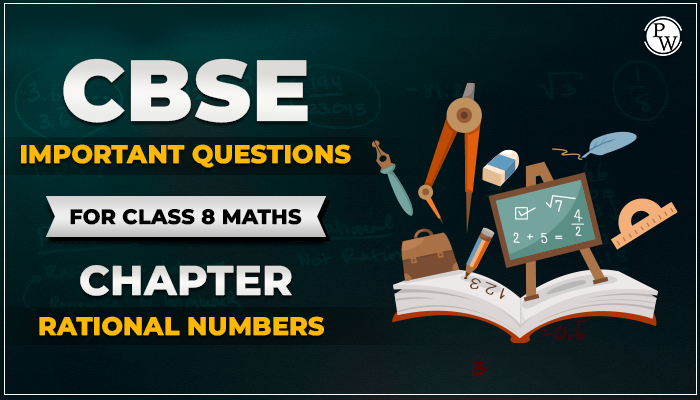# CBSE Important Questions for class 8 Maths chapter Rational Numbers

#### Important Questions for CBSE Class 8 Maths

This page is prepared by academic team of Physics Wallah. Download Important Questions for Class 8 Maths Chapter 1 Rational Numbers free Pdf. The pdf of chapter 1 Rational Numbers consists of step-by-step solutions of all questions.

Physics Wallah is a platform having Expert teacher pool from school and coaching institution who prepared NCERT Solutions and other resource material. Solve question given in Exercise of NCERT text book with the help of NCERT solutions for Class 8 Maths. For additional information related to the subject you can check the Maths Formula section. Do solve maths subject CBSE Sample papers for Class 8 Maths prepared by Physics Wallah.### Short Answer Type Questions

• Q1. What are the multiplicative and additive identities of rational numbers?
• Ans. 0 and 1 are rational numbers' additive and multiplicative identities.
• Q2. Write the additive inverse of 19/-6 and -2/3
• Ans. 19/-6 = 19/6 and -⅔ = 2/3
• Q3. Write the multiplicative inverse of -13/19 and -7
• Ans. -13/19 = -19/13 and -7 = -1/7
• Q4. Mention a rational number that has no reciprocal.
• Ans. A rational number “0” has no reciprocal or multiplicative inverse.
• Q5. Mention any 4 rational numbers which are less than 5.
• Ans. -1, 1, 2 and 3.

### Long Answer Type Questions

• Q6. Mention the commutativity, associative and distributive properties of rational numbers. Also, check a × b = b × a and a + b = b + a for a = 1/2 and b = 3/4
• Ans. Commutative property:
• For any two rational numbers, a and b, a + b = b + a.
• For any two rational numbers, a and b, a × b = b × a.
• Associative Property:
• For any three rational numbers a, b and c,
• (a + b) + c = a + (b + c)
• Distributive property states that for any three numbers x, y and z,
• x × ( y + z ) = (x × y) + ( x × z)
• a x b = b x a
• a x b = 1/2 x 3/4 = 3/8
• b x a = 3/4 x 1/2 = 3/8
• a + b = 3/4 + 1/2 = 5/4
• b + a = 1/2 + 3/4= 5/4
• Q7. Write any 5 rational numbers between −2/5 and ½.
• Ans. −2/5 can be written as −8/20.
• 1/2 can be written as 10/20.
• So, rational numbers between these two numbers can be,
• −7/20,−6/20,−5/20,−4/20,−3/20,−2/20,−1/20,0,1/20,2/20,3/20,4/20.
• Q8. If the product of any two rational numbers is two and one is 1/7, find the other?
• Ans. Consider two rational numbers: "a" and "b."
• Given, a = 1/7 and a × b = 2
• Now, 1/7 × b = 2
• b = 7 × 2 = 14
• So, the other rational number will be 14.
• Q9. Mr. X went shopping with a certain amount of money. He spent Rs. 10(¼) on buying a pen and Rs. 25(¾) in food. He then gave the remaining Rs. 16(½) to his friend. Calculate how much money he initially had.
• Ans. To get the amount of money Mr. X initially had, his purchases must be added.
• So,
• Initial Money = 10(¼) + 25(¾) + 16(½)
• = 41/4 + 103/4 + 33/2
• By taking LCM, we get
• Initial Money = 105/2

#### Find below Important Questions for class 8 Maths chapter 1 Rational Numbers